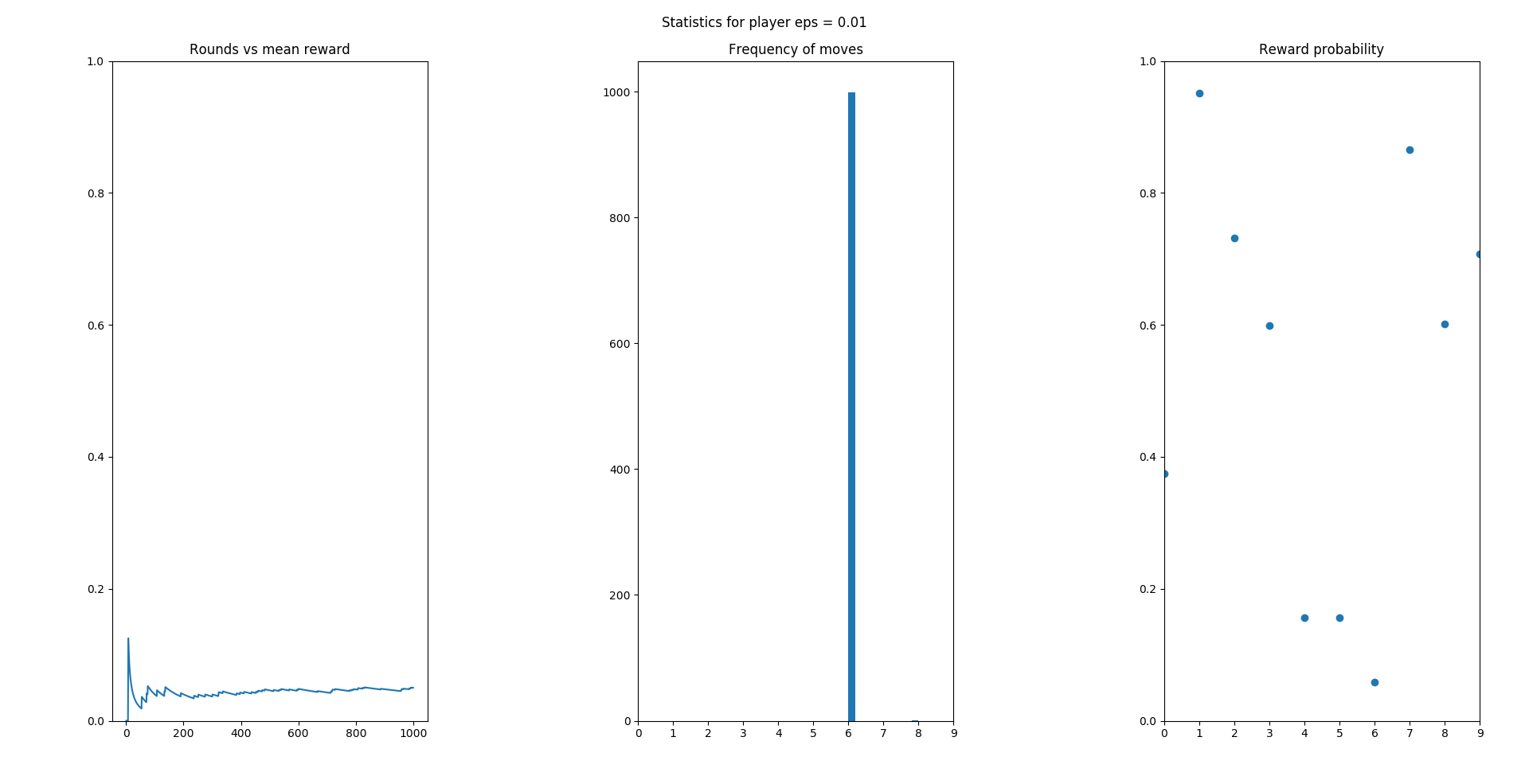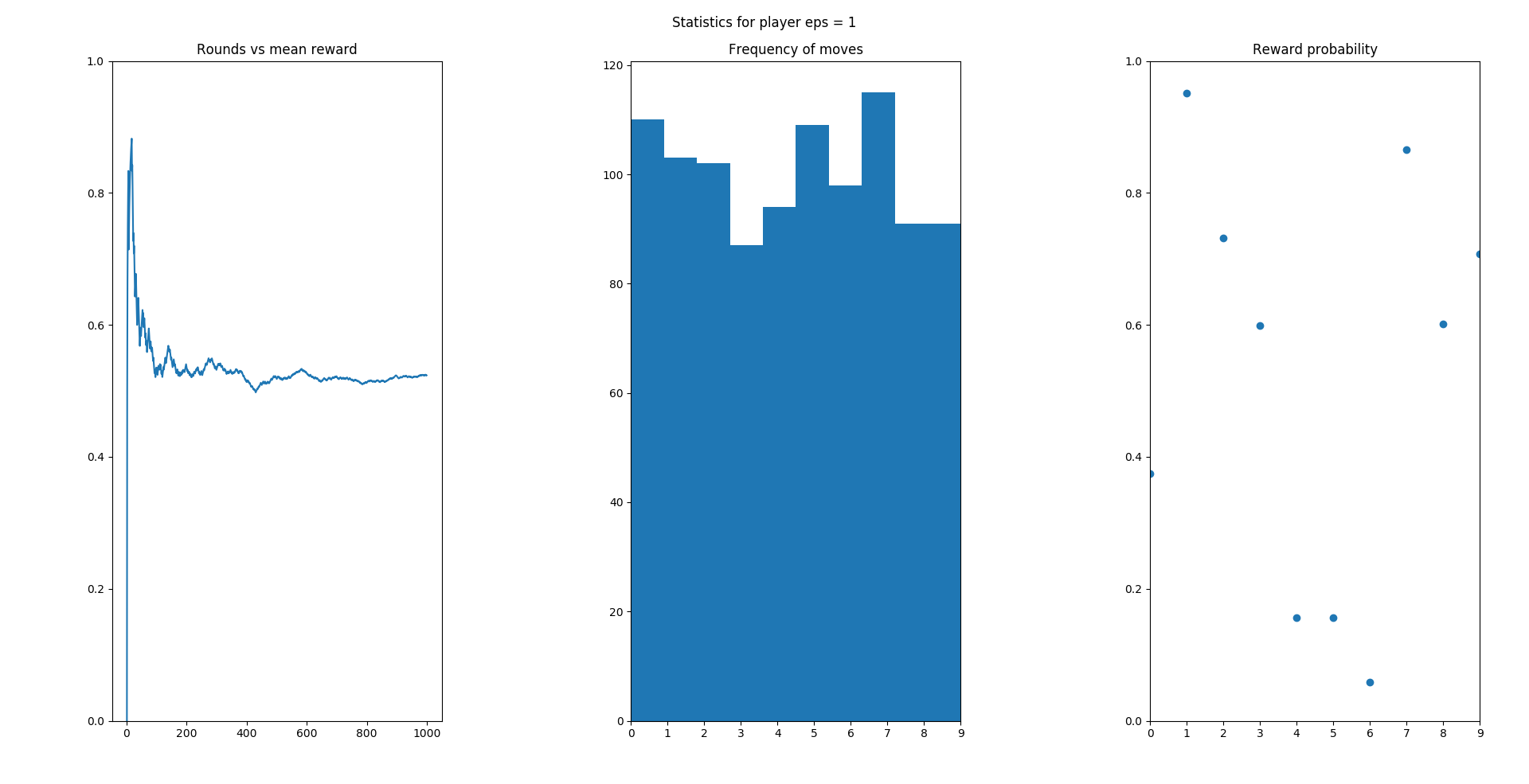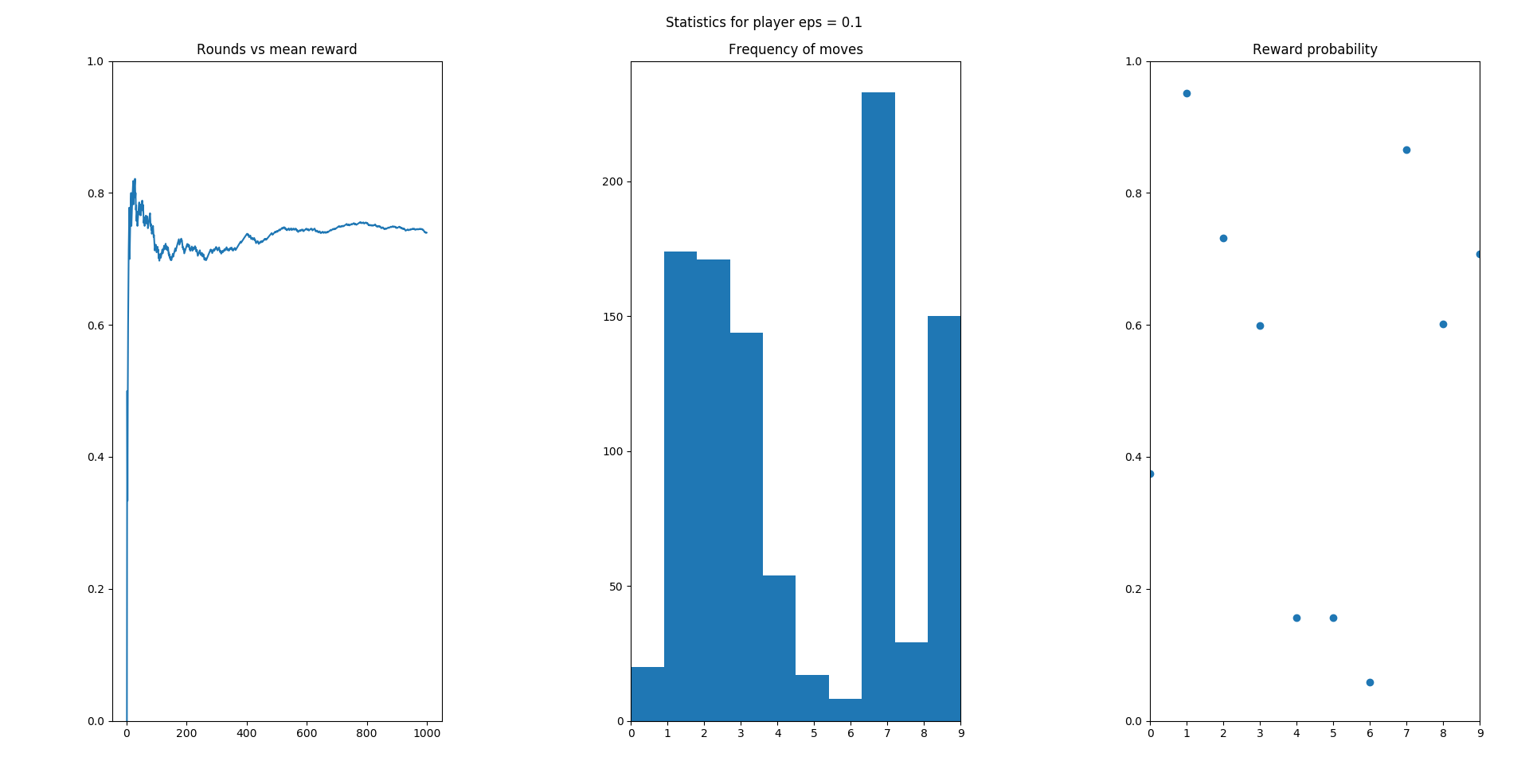The $$k$$ armed bandit problem can be phrased as follows:

• There are $$k$$ possible actions, $$\{a_1, a_2, \ldots , a_k\}.$$ Actions are sometimes called arms (hence $$k-$$ armed).
• At time $$t = 1, 2, \ldots ,$$ an action is chosen and a reward $$R_t$$
is allocated.
• Each action gives an unknown reward, sampled from an unknown
probability distribution.
• The reward depends only on the action.
• Goal: Maximize total reward.

These are a simpler class of reinforcement learning problems on which there is no influence from the state. Our actions have a noisy effect in the reward and our goal is to learn from these noisy signals. However, the effect of our actions on the reward is not influenced by any other externalities.

Bandit algorithms are useful in a number of applications:

• Personalized content/news.
• Online advertising (A/B testing and similar). You have probably heard of A/B testing. A/B testing is a bandit algorithm with a pure exploration phase, followed by pure exploitation. Don’t worry, this will be clear soon.
Clinical trials (experimental treatments for patients while
minimizing losses).
• Portfolio Optimization.

### Solving bandit algorithms

Let’s figure out how to deal with bandits before moving on to harder reinforcement learning problems.

As we said for every action $$a_i$$, the reward might be still random, but it only
depends on the action.

$$q_*(a_i) := \mathbb E \left[ R_t \ | \ A_t = a_i \right], \ \ i = 1, 2, \ldots ,k.$$

To maximize rewards, we create some estimates of the $$q_*$$ function
above, $$Q_t(a_i) := q_*(a), \ i = 1, 2, \ldots k$$ and use those estimates to choose the best actions. The $$q_*$$ function is the best possible return we could get from each action, whereas $$Q_t$$ represents our current estimate of the $$q_*$$ function based on what we just learned from the interaction.

One way to learn about $$q_*$$ is to keep track of the average rewards.

$$Q_t(a_i) := \frac{\text{total reward of } a_i \text{ before } t}{\text{times } a_i \text{ was played time } t} = \frac{ \sum_{j=1}^{t-1}R_j\cdot 1_{A_j=a_i}}{\sum_{j=1}^{t-1} 1_{A_j=a_i}}.$$

By the law of large numbers, if the action is taken infinitely many times,

$$\lim_{N_t(a_i) \to +\infty} Q_t(a_i) = q_*(a_i).$$

### Exploration vs Exploitation

A dilemma that arises in many decision problems is the trade-off between exploration and exploitation. This simply means that if we spend a lot of time trying things randomly, we are risking our reward in the long term, out of low commitment to well-performing actions. Conversely, sticking to something too soon might fire back: imagine we try 2 out of 10 different arms of our bandit, and we just stick to the best of those two. We might be ignoring a much better arm if we don’t get adventurous and try it! When we just commit to such action (i.e. the best action seen so far) we say we use a greedy policy.

• The greedy policy at time $$t$$ is choosing actions $$A_t^*$$ such
that:
$$A_t^* \ \in \ \mathrm{argmax}_{i = 1, 2, \ldots ,k} Q_t(a_i).$$
• If at time $$t$$ we choose action $$A_t$$ such that $$A_t = A_t^*$$ is exploiting the action, whereas
$$A_t \neq A_t^*$$ means exploring.

One way to deal with the exploration-exploitation trade-off is to be $$\epsilon$$-greedy

• Create an action-value table (a Python dictionary) storing the
history of the payoffs obtained by each action.
• Calculate the average payoff of each action.
• Choose with probability $$\epsilon$$ a non-optimal action.
• Choose with probability $$1-\epsilon$$ one of the greedy actions
(those with highest average payoff).
• Break ties randomly.

The choice of $$\epsilon$$ is important, and we can observe different types of behaviour.

The figures below illustrate the rewards an agent gets for choosing different arms with different reward probabilities (shown in the third image from the right). The reward is 1 when you get it. So we see that the very conservative player (with small $$\epsilon$$ get stuck with a single action from the beginning.On the opposite behaviour, with a value of $$\epsilon=1$$, we see a player that does a bit better and explores a wider range of actions (in the second picture). However, the mean reward is barely around the average. This is because he is just playing randomly.Finally, we have a more balanced agent, which gets a much higher reward in average because is able to identify the correct actions to use with time.Interested? Check out Part 2! Or the book in Leanpub.